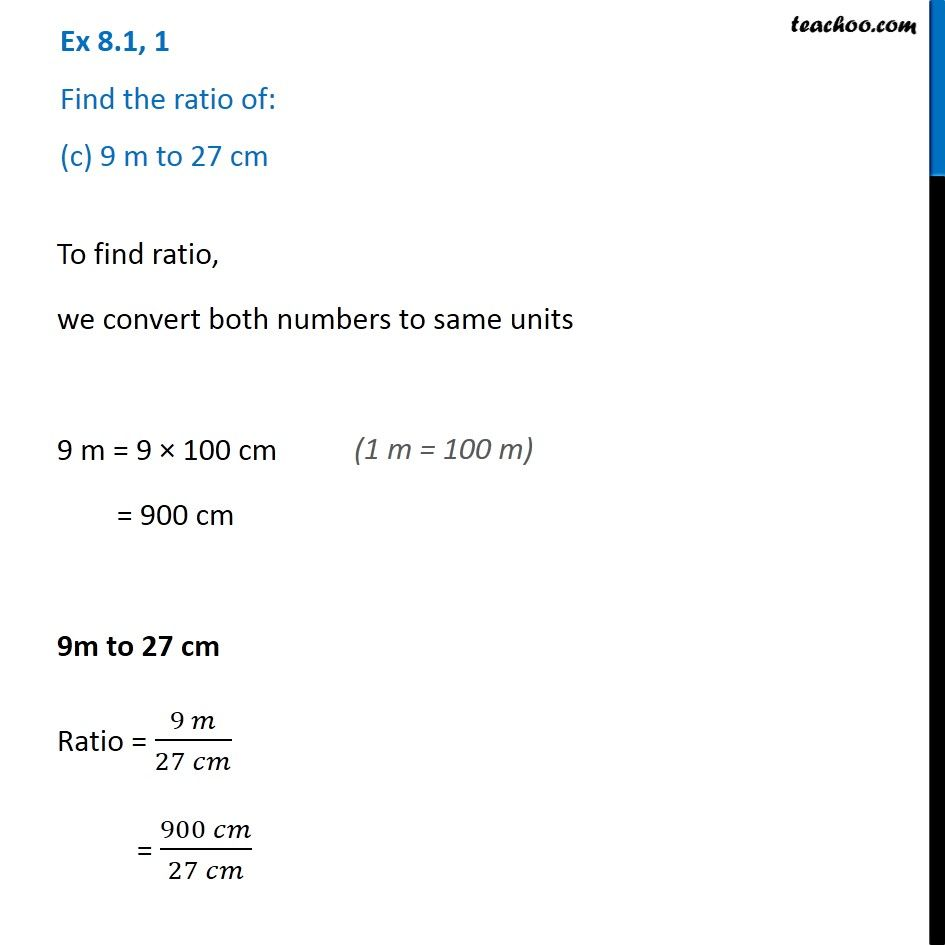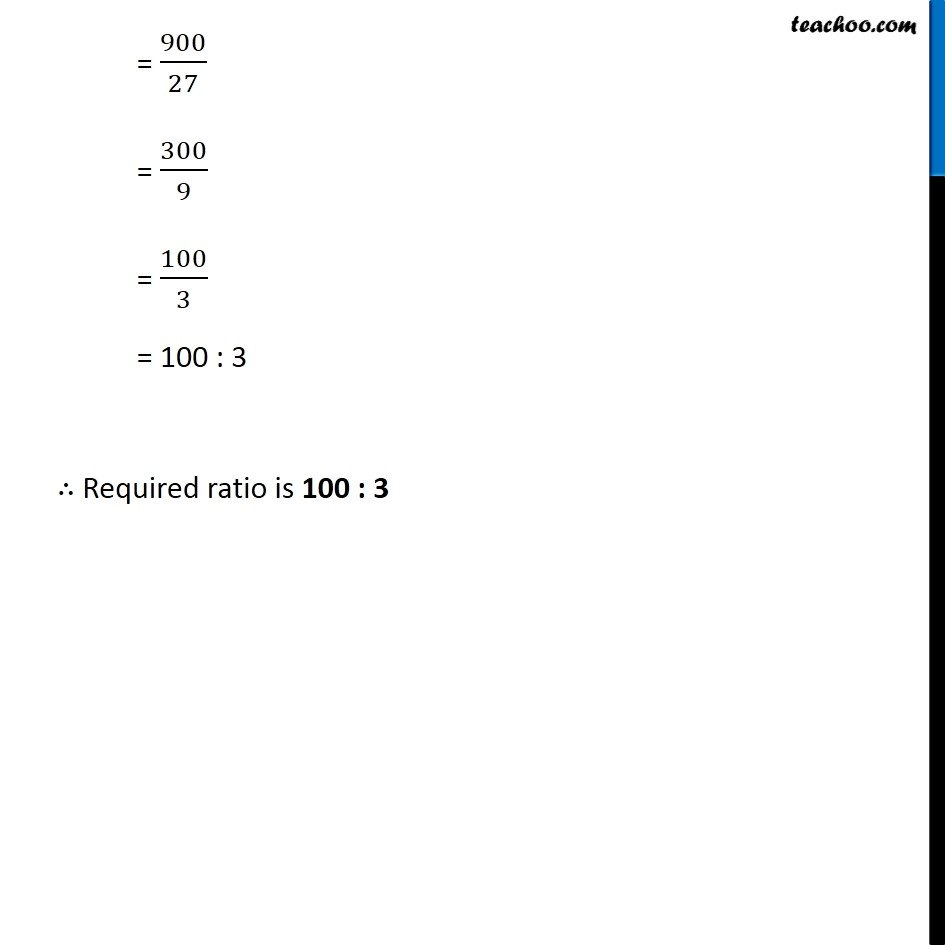Finding Ratios

Chapter 7 Class 7 Comparing Quantities
Serial order wiseLearn in your speed, with individual attention - Teachoo Maths 1-on-1 Class

### Transcript

Question 1 Find the ratio of: (c) 9 m to 27 cm To find ratio, we convert both numbers to same units 9 m = 9 × 100 cm = 900 cm 9m to 27 cm Ratio = (9 𝑚)/(27 𝑐𝑚) = (900 𝑐𝑚)/(27 𝑐𝑚) = 900/27 = 300/9 = 100/3 = 100 : 3 ∴ Required ratio is 100 : 3